# Taking Dielectric Measurements to the Liquid State

May 25, 2021 by Meng Li

Liquid dielectrics are dielectric materials in a liquid state and are widely used in electrical insulation as well as cooling. Nonetheless, their fluidity also makes accurate dielectric measurements a challenge. In a previous blog post, we saw how to measure solid-state materials with the MFIA Impedance Analyzer and a pair of parallel metal-plate electrodes as the testing fixture. Measurements in the liquid state can be carried out in a very similar way. Here, we will present how to perform liquid dielectric spectroscopy.

## Experiment Setup

The setup includes the MFIA and a third-party liquid dielectric fixture, whose top and the bottom electrodes are wired to the Hcur and Lcur ports on the MFIA, respectively, as shown in Figure 1. The diameter (d) of the guarded bottom electrode is 2 cm. The thickness (t) of the liquid dielectric sample can be finely adjusted by lowering the top electrode which is connected to a digital micrometer.  In this configuration, the typical volume is 1 mL or less. Assuming the liquid can wet metal electrode plates, surface roughness is not an issue anymore. However, leakage current may become a concern at low frequencies. Please read on for more details.

The measured liquid dielectric sample in this blog post is olive oil. Note that different brands can have very different constituents, so results may differ.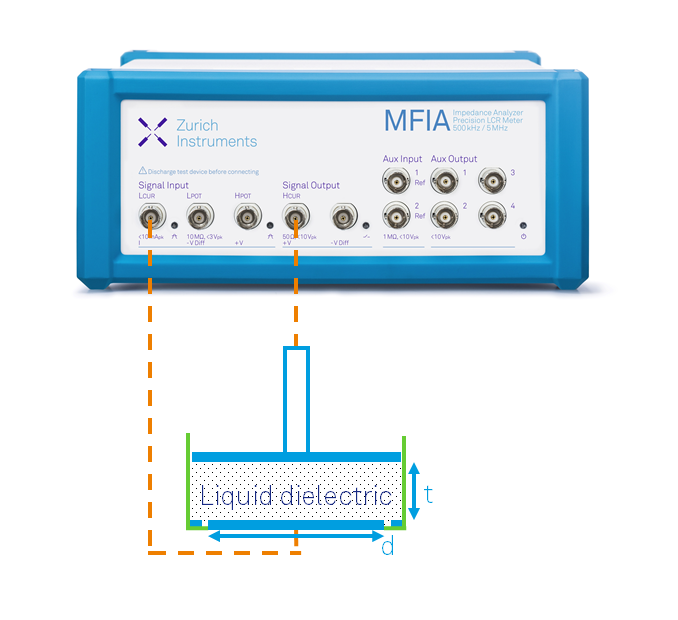Figure 1: The MFIA Impedance Analyzer is connected to a third-party liquid dielectric test fixture in a 2-terminal configuration. The position of the top electrode can be adjusted with a digital micrometer, giving a sample thickness of t. The bottom electrode has a diameter equal to d.

### Measurement using default Sweeper settings

We use the default Sweeper settings as a convenient starting point. As most dielectric samples are highly resistive, we choose a 2-terminal measurement with a test signal of 1 V in the LabOne® software to maximize the current. Figures 2 and 3 show the measured results of 1-mm-thick (in red) and 3-mm-thick (in blue) samples between 0.1 Hz and 5 MHz.

Thanks to the 8-stage built-in current input on the MFIA, even a tiny amount of current (pA range) can be detected. As a result, we can easily measure even above 400 GOhm at 0.1 Hz. As expected, the 3-mm-thick sample shows 3 times as large impedance as the 1-mm-thick sample. We also notice that, in both cases, the impedance decreases linearly (a slope of -1 on a log-log scale). In contrast, the phase is similar. But given the weak current signal, it is no surprise that the thicker sample with less current is noisier. In addition, we find that the phase is not -90 deg at low frequencies, deviating significantly from a pure capacitor. This strongly hints that there are additional parasitic impedances in the fixture, and a two-element equivalent circuit model may not be complete here.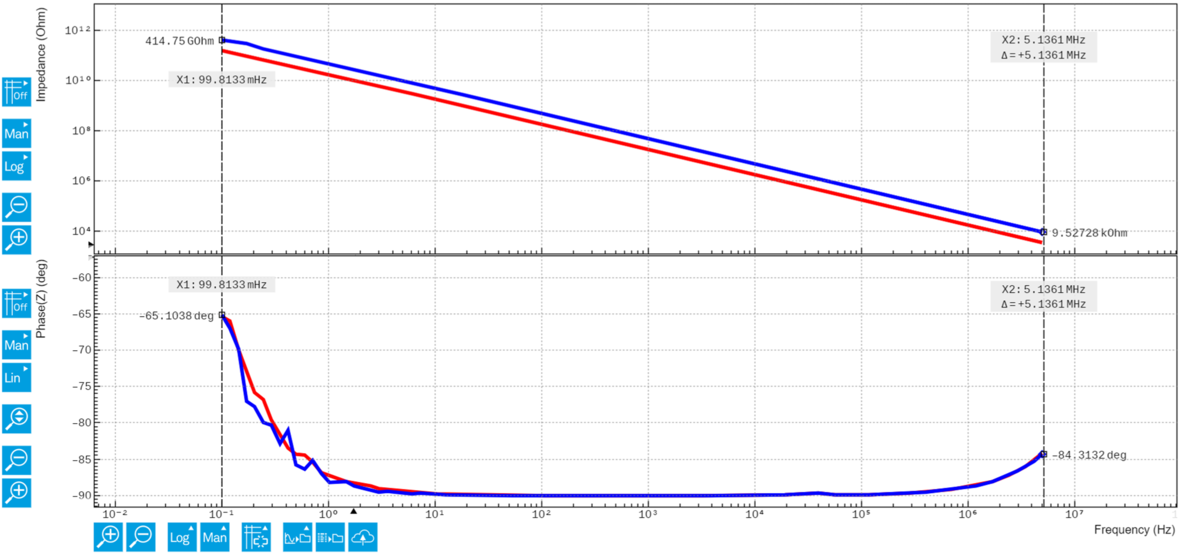Figure 2: Screenshot of LabOne Sweeper module showing impedance amplitude (top) and phase (bottom) from olive oil with different thicknesses.

For now, let's proceed with the built-in D Cp or D Cs model in LabOne to extract the capacitance C. The derived C is flat over the entire frequency span. Taking the value (9 pF) from the 1-mm-thick sample, we can calculate a relative dielectric constant (permittivity) of 3.23 for the olive oil.

On the other hand, the dissipation factor (loss) D reveals a completely different trend. Since D is a material property that does not change with dimension, it remains the same in the 2 different samples. However, D does not stay as a constant with respect to frequency. It decreases to a minimum of 2x10-4 at 1 kHz, and then rises again almost linearly until 5 MHz. There is also a step at 50 kHz, due to the current input range switching in auto-ranging mode. This step in phase is in fact very small on a linear scale, as shown in Fig. 2. It is just visually 'amplified' when we look at the D (inverse 'tangent' of the absolute phase), plotted on a log scale.

To fully understand this behavior, we will need to delve a bit into circuit modeling, using third-party programing language or analysis software. The details can be found in the final section of the blog post.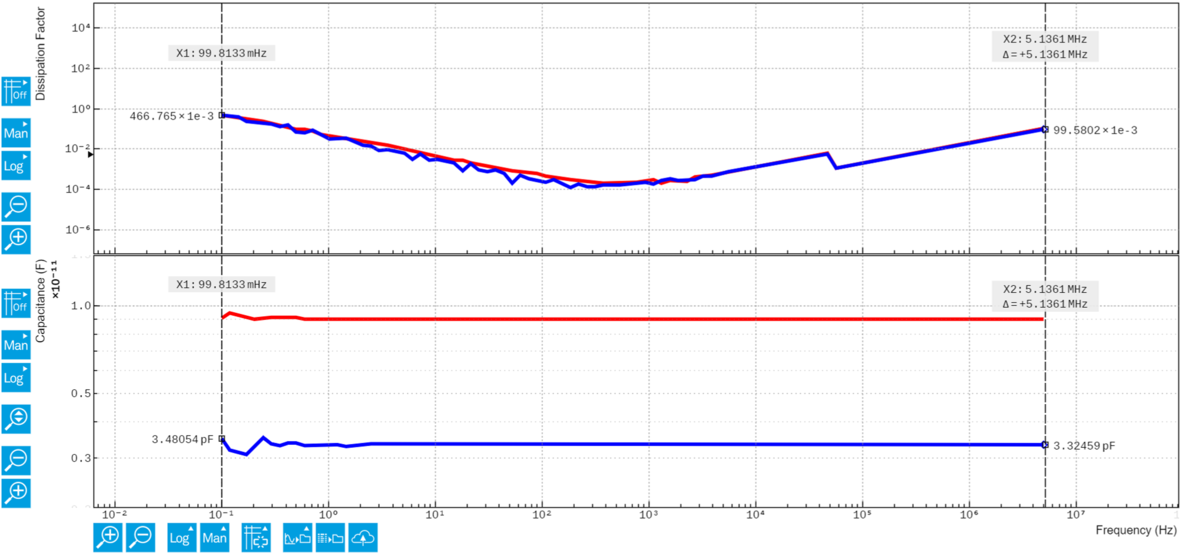Figure 3: Screenshot of the LabOne Sweeper module showing the derived D (top) and C (bottom) from olive oil with different thicknesses.

### Measurement using manual Sweeper settings

To prevent the step due to the range switch at around 50 kHz, we can use a manually fixed current input range at 1 mA. Naturally, this means the current will be limited by the bit resolution of the analog-digital converter (ADC) at the current input. So rather than a full range sweep, we only run the measurements starting from 10 kHz. This frequency region is also suitable for using two-element circuit models such as D Cs or D Cp.

As the current is very small (400 nA in 1 mA range) at 10 kHz, measuring a phase so close to -90 deg is difficult. Figure 4 shows the result using the default maximal low-pass filtering bandwidth at 100 Hz in the red trace. The derived D is noisy, and also discontinuous on the log scale (slightly exceeding -90 deg). To solve this problem, we can lower the bandwidth to 1 Hz,  in the advanced settings in the sweeper tab. In doing so, the measurement speed is decreased, but we can obtain a smoother result (the blue trace). The minimal D measured here is likely limited by the fixture, and it should be mentioned that the MFIA can measure an even lower D (1x10-5) in high-Q ceramic samples. You can find more relevant information in an earlier blog post.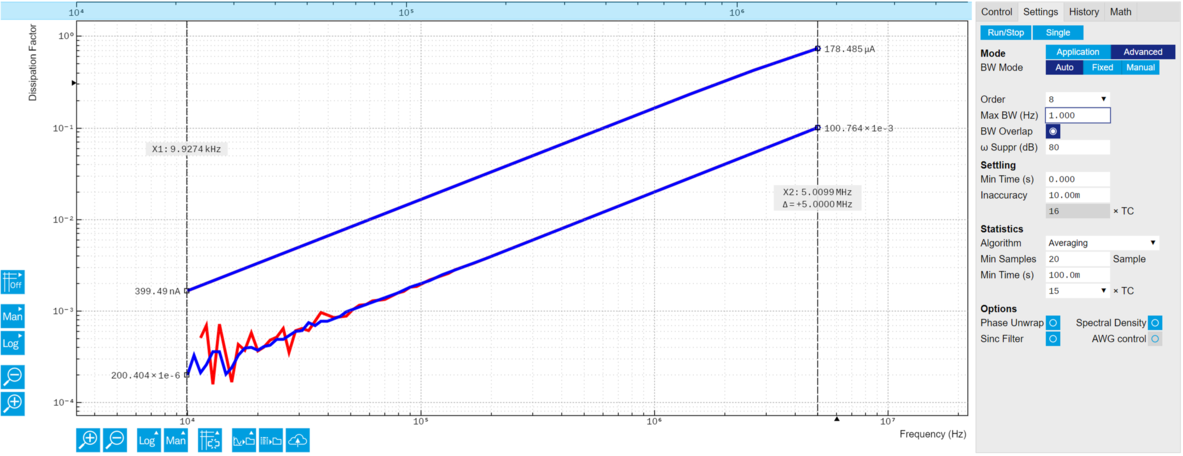Figure 4: Screenshot of the LabOne Sweeper module showing the measured (RMS) current and D on a log-log scale. The red trace shows a measurement with a default 100 Hz low-pass filtering bandwidth. The smoother blue one is achieved with 1 Hz. A black square highlights where the settings can be changed.

## Understanding the Measurements at Low Frequencies

Why can we not reach such a low D in our measurements at low frequencies? As briefly mentioned above, parasitic impedance plays a key role here. For simplicity, let's just consider a three-element circuit model, R1+(R2||C1), by adding a parasitic resistance R2 (345 GOhm, due to leakage current) to the R1+C1 model (the latter is equivalent to D Cs). As such, at low frequencies where the impedance of R2 is less than C1, the current will bypass C1. As we approach DC, we won't see any capacitive effects at all.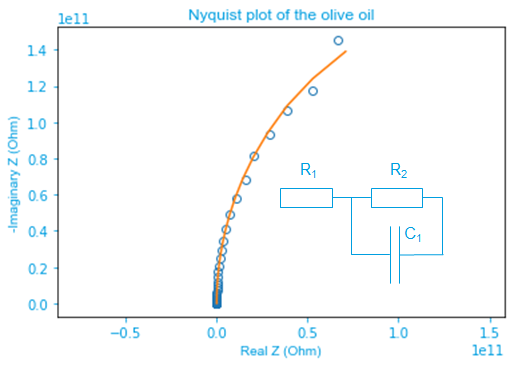Figure 5: Nyquist plot showing the measured (blue open dots) and fitted (orange solid line) impedance spectra of the olive oil sample. The fitting assumes a three-element R1+(R2||C1) equivalent circuit model. Fitting and replotting were done in Python.

At high frequencies (>10 kHz), C1 becomes low in impedance. Only then can the circuit be reduced into R1+C1 (or D Cs). Taking the fitted R1 (357 Ohm) and C1 (9.08 pF), we can calculate D using the equation below. This equation indeed predicts a linear dependence on the frequency f, as we see in Figure 5. The value of D (0.1) at 5 MHz in the plot also confirms the equation.

$D=2\pi fR_{1}C_{1}$

## Conclusion

In this blog post, we described how to measure dielectric spectra in liquid dielectrics. Using the MFIA, we can accurately measure olive oil samples with capacitances at pF level. The results show a dielectric constant of 3.23. Regarding the dissipation factor, we find it to be linearly proportional to the frequency. The relationship agrees well with a three-element R+(R||C) circuit model.

If you are interested in this type of measurement, please get in touch with us to set up a demo.